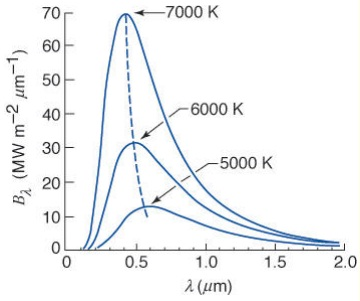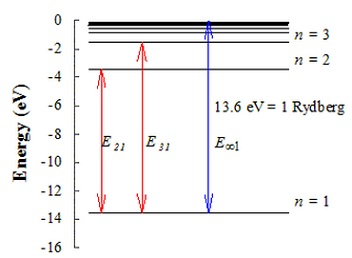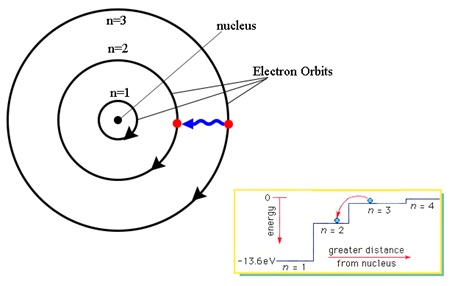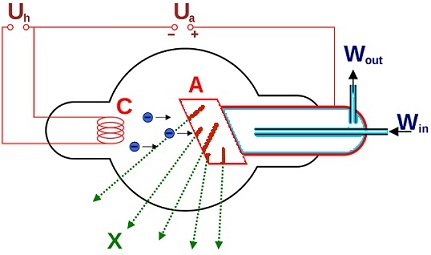#### Modern Physics, Physics tutorial

Introduction to Modern physics:

The progress in Physics from the 17th to 19th century arrive its pinnacle towards the end of the 19th century. The Newtonian model of synthesizing various branches like Heat, Mechanics, Sound, Electricity, Magnetism and Optics come out to be at its peak. Though, three observations were defying this model, the black body radiation spectrum, Balmer's formula for line Spectrum of Hydrogen Atom and Michelson- Morley's null outcomes were challenges to the very basics of Newtonian concepts of Space, Time and Mass on one hand and Newton's motion of determinism on the other.

The resolution to such 'three dark clouds' according to the Lord Kelvin, opens up a new period in Physics since the starting of the 20th Century, the generic word for subsequent growths is Modern Physics and in dissimilarity, Physics, up to the 19th Century is termed as Classical Physics.

Photon and the Atom:

Blackbody Radiation and the Quantum Theory:

A blackbody is a surface which:

a) Completely absorbs all the incident radiation.

b) Emits radiation at the utmost possible monochromatic intensity in all directions and at all wavelengths.

The concept of the energy distribution of blackbody radiation was introduced by Planck and first appeared in the year 1901.

Planck hypothesized that energy can be absorbed or emitted only in discrete units or photons having energy:

E = hν

The constant of proportionality is h = 6.626 × 10-34 J s.

Planck represented that the intensity of radiation emitted through a black body is represented by:

Bλ = c1λ-5/exp (c2/λT) - 1

Here c1 and c2 are constants

c1 = 2πhc2 = 3.74×10-16 Wm-2

c2 = hc/k = 1.44×10-2 mK

The function Bλ is termed as the Planck function.

The Blackbody radiation is isotropic.

If Bλ(T) is plotted as a function of wavelength on the linear scale the resultant spectrum of monochromatic intensity represents the shape described as shown below:Blackbody emission (that is, the Planck function) for absolute temperatures as pointed is plotted as a function of the wavelength on a linear scale.

Photon Energy, Momentum and Wavelength:

A beam of electromagnetic radiation, regarded as an electromagnetic wave, is characterized through its frequency, 'f' or its wavelength 'λ' that are associated by:

f = c/ λ

The similar beam, regarded as a stream of photons, is characterized by the energy 'E' or the momentum 'p' of the individual photons. A photon consists of no mass and travels at a speed, c = 3.00 x 108ms-1. Its energy and momentum are associated by:

E = cp

The fundamental connecting link between such two explanations of the similar beam of radiation, proposed by Einstein, is that the photon energy 'E' is proportional to the frequency 'f' of the electromagnetic wave.

E = hf

The constant of proportionality 'h' is the Planck's constant.

The Electromagnetic radiation can be categorized according to the energy of its photons, or the wavelength, or the frequency, whichever is most suitable.

For instance, from f = cλ, the above equation can be written to represent the relationship between the photon energy 'E' and wavelength:

E = hc/λ

Photon energies are generally specified in electron volts, and wavelengths in the angstrom unit. Inserting the numerical values of 'h' and 'c' and the requisite conversion factors, we write the equation in a form that is handy for computations:

E = (12.4 x 103AoeV)/λ

The above provides the photon energy 'E' in electrovolts if the wavelength 'λ' is in angstrom units.

The Nuclear Atom:

The model of the atom in the Newton's day was that of a small, hard indestructible sphere. This model was a good base for the kinetic theory of gases. Though, new models had to be devised if experiments revealed the electrical nature of atoms. The discovery of the electron in the year 1897 prompted J.J. Thomson (1856 - 1940) to propose a new model of the atom. In Thomson's model, electrons are implanted in a spherical volume of positive charge similar to seeds in a watermelon.

In the year 1911 a planetary model, in which the electrons revolve similar to planets round a small, massive, positively charged nucleus resulted from the Rutherford's experiments on the scattering of α-particles through gold or platinum foil.

The nucleus is now assumed to comprise of protons of mass 1 and charge + 1e, and neutrons of mass 1 and charge 0. Surrounding the nucleus is planetary electrons of mass 1/1840 and charge   - 1e. The structure of the three lightest elements is as shown:

 Elements Nucleus Planetary Electrons Hydrogen Charge 1 mass 1 (1 proton) 1 Helium Charge 2 mass 4 (2 protons and 2 neutrons) 2 Lithium Charge 3 mass 7 (3 protons and 4 neutrons) 3

The atoms of the remainder of the elements are made in an identical manner. The atomic number provides the charge on the nucleus and as well the number of planetary electrons; the atomic mass minus the atomic number provides the number of neutrons in the nucleus.

The Bohr Atom:

In the year 1913 Bohr introduced his quantized shell model of the atom to describe how electrons can have stable orbits about the nucleus. The motion of the electrons in the Rutherford model was unstable as, according to the classical mechanics and electromagnetic theory, any charged particle moving on the curved path emits electromagnetic radiation; therefore, the electrons would lose energy and spiral into the nucleus. To cure the stability trouble, Bohr altered the Rutherford model by needing that the electrons move in orbits of fixed energy and size. The energy of an electron based on the size of the orbit and is lower for the smaller orbits. Radiation can take place merely if the electron jumps from one orbit to the other. The atom will be fully stable in the state by the smallest orbit, as there is no orbit of lower energy to which the electron can jump.

The emitted wavelengths were early on related by a set of discrete energy levels En represented by:

En = - 13.6 eV/n2, with n = 1, 2...

as the emitted photon energies equivalent the energy difference discharged if an electron forms a transition from a higher energy Ei to a lower energy Ej.

Eph = 13.6 eV (1/j2 - 1/i2), with i > j

The maximum photon energy emitted from the hydrogen atom equivalents 13.6 eV. This energy is as well termed as one Rydberg or one atomic unit. The electron transitions and the resultant photon energies are further described by the figure shown below:Niels Bohr gave a part of the puzzle. He supposed that electrons move all along a circular trajectory about the proton similar the earth around the sun, as represented in figure shown below:He as well supposed that electrons act in the hydrogen atom as a wave instead of a particle. Thus, the orbit-like electron trajectories around the proton are limited to such having a length that equals an integer number of wavelengths in such a way that:

2πr = n λ

Here, 'r' is the radius of the circular electron trajectory and 'n' is a positive integer. The Bohr model as well supposes that the momentum of the particle is connected to the de-Broglie wavelength.

The model further supposes a circular trajectory and that the centrifugal force equivalents the electrostatic force or:

mv2/r = q2/4πεor2

While solving for the radius of the trajectory one finds out the Bohr radius, a0:

ao = εoh2n2/πmoq2

and the corresponding energy is acquired by adding up the kinetic energy and the potential energy of the particle, resulting:

En = - moq4/8εo2h2n2, having n = 1, 2.....

Here, the potential energy is the electrostatic potential of the proton:

V(r) = -q2/4πεor

X-rays:

X-ray Spectra:

X-rays are the form of electromagnetic radiation containing short wavelengths and high frequencies, around 1018 - 1019 Hz.

Their production can be described by employing the energy levels theory, as X-rays are generated by streams of high-energy electrons in collision having atoms of high atomic number, like tungsten or molybdenum.

X-rays are generated, by two distinct methods if the electron strikes a metal target.

1) The electrons all of a sudden lose energy if they collide by the target nuclei. A big percentage of the energy is transformed into heat; however a few is transformed into X-ray photons. Each and every electron gains the similar energy from being accelerated through the tube voltage, however varying fractions of this energy are transformed into photons. As usual, the energy of the photon created = hf. The maximum frequency (or minimum wavelength) will be generated if all the energy gained by an electron is transformed into a photon. A continuous range of smaller frequencies (or greater wavelengths) is formed by smaller fractions of the electrons energies being transformed into photons. This continuous X-ray spectrum is typical of the tube voltage however independent of the target material.

2) The electron provides a few of its energy to an electron in the target atom. The electron in the atom jumps up to the higher level and X-rays are emitted as the electron falls back to the lower level. The energy 'hf' of the photons generated is equivalent to the energy level difference. The energy of an X-ray photon is extremely large, and thus the energy levels comprised should encompass a large separation. The frequencies (and wavelength's) emitted contain discrete values that are typical of the target material. This gives increase to the peaks.

X-ray Tube:The figure above represents the necessities of the x-rays tube introduced by Coolidge in the year 1913. The tube is exhausted as highly as possible in such a way that no discharge would pass via it if it were employed as a gas tube. The electrons are emitted through a tungsten filament 'C' and the rate of their emission, which finds out the intensity of the X-rays, can be controlled through the heating current via the filament.

The hardness of the X-rays is regulated separately by the potential difference between A and C. Their hardness based on their frequency and if it is greater the faster the speed of the electrons striking the target. The phenomenon is a type of reverse photoelectric effect and an identical relation holds:

Ve = hf

Here 'V' is the potential difference via which the electrons fall, 'e' is the charge of the electron, 'h' is the Planck's constant and f is the maximum frequency of the X-rays emitted.

Nature and Properties of X-rays:

X-rays are the electromagnetic waves, similar to light, having the similar speed of 3 x 108ms-1, however having a wavelength about a thousand times shorter than that of the light waves. Their wavelengths range from 0.06 to 100 Angstrom units against 3,900 to 7,600 Angstrom units of visible light.

1) X-rays travel in the free space having a speed of 3 x 108ms-1, the same that of light.

2) They influence a photographic plate much more intensely than light due to their very much shorter wavelength.

3) They are not influenced through magnetic and electric fields, clearly representing that they are not a stream of the charged particles.

4) Similar to light, they release photoelectrons if allowed to fall on some particular metals.

5) Scattered X-rays represent a marked degree of polarization similar to the scattered sky light.

6) They experience reflection, refractions, dispersion and diffraction.

7) They can ionize a gas (or air) via which they are permitted to pass.

8) They cause fluorescence in substances such as barium plastinocyanide, zinc sulphide and cadmium tun state.

Uses of X-rays:

The worth of X-rays is mostly due to their penetrating power:

1) Medicine: Radiographs or X-ray photographs are employed for a diversity of purposes. As illustrated earlier, X-rays can pass via flesh however not via bones. Thus, sharp dark shadows of the bony parts of the body are acquired against a lighter background on a fluorescent screen or a photographic plate, when these are interposed in the path of the Xx-rays. These X-ray photographs are termed as radiographs. Dislocation, fractures and the presence of foreign bodies such as bullets, pins and so on within the human body can therefore be with no trouble detected. In radio therapy, periodic X-ray exposures, in appropriately controlled doses, are given for the treatment of obstinate skin diseases and malignant or cancerous growths or tumors.

2) Industry: Casting and welded joints can be examined for the internal imperfections by employing X-rays. A complete machine might as well be inspected from the radiograph without having to be taking apart.

3) X-ray crystallography: The study of crystal structure through X-rays is now a powerful technique of scientific research. The first crystals to be analyzed were of simple compounds like sodium chloride however in present years the structure of extremely complex organic molecules has been unraveled.

Tutorsglobe: A way to secure high grade in your curriculum (Online Tutoring)

Expand your confidence, grow study skills and improve your grades.

Since 2009, Tutorsglobe has proactively helped millions of students to get better grades in school, college or university and score well in competitive tests with live, one-on-one online tutoring.

Using an advanced developed tutoring system providing little or no wait time, the students are connected on-demand with a tutor at www.tutorsglobe.com. Students work one-on-one, in real-time with a tutor, communicating and studying using a virtual whiteboard technology.  Scientific and mathematical notation, symbols, geometric figures, graphing and freehand drawing can be rendered quickly and easily in the advanced whiteboard.

Free to know our price and packages for online physics tutoring. Chat with us or submit request at info@tutorsglobe.com